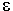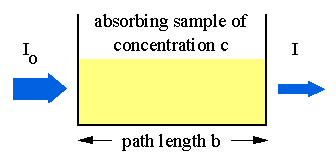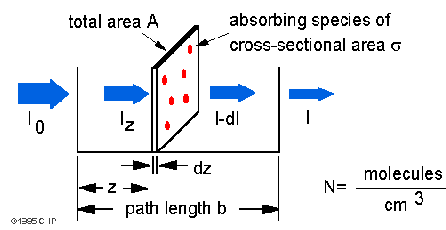# Beer-Lambert Law

### Introduction

The Beer-Lambert law (or Beer's law) is the linear relationship between absorbance and concentration of an absorbing species. The general Beer-Lambert law is usually written as:

A = a() * b * c

where A is the measured absorbance, a() is a wavelength-dependent absorptivity coefficient, b is the path length, and c is the analyte concentration. When working in concentration units of molarity, the Beer-Lambert law is written as:

A =* b * c

whereis the wavelength-dependent molar absorptivity coefficient with units of M-1 cm-1. Data are frequently reported in percent transmission (I/I0 * 100) or in absorbannce [A = log (I/I0)]. The latter is particularly convenient. [common coefficients of near-ultraviolet absorption bands of some amino acids and nucleotides]

Sometimes the extinction coefficient is given in other units; for example,

A = E1% * b * c

where the concentration C is in gram per 100 ml of solution. This useful when the molecular weight of the solute is unknown or uncertain.

Q: Cytosine has a molar extinction coefficient of 6*103 at 270 nm at pH 7. Calculate the absorbance and percent transmission of 1*10-4 and 1*10-3 M cytosine solution in a 1-mm cell. [Solution....]

Q: A protein with extinction coefficient E1% = 16 yields an absorbance of 0.73 when measured in a 0.5-cm cell. Calculate the weight concentration. [Solution....]

### Instrumentation

Experimental measurements are usually made in terms of transmittance (T), which is defined as:

T = I / Io

where I is the light intensity after it passes through the sample and Io is the initial light intensity. The relation between A and T is:

A = -log T = - log (I / Io).

Absorption of light by a sampleModern absorption instruments can usually display the data as either transmittance, %-transmittance, or absorbance. An unknown concentration of an analyte can be determined by measuring the amount of light that a sample absorbs and applying Beer's law. If the absorptivity coefficient is not known, the unknown concentration can be determined using a working curve of absorbance versus concentration derived from standards.

### Derivation of the Beer-Lambert law

The Beer-Lambert law can be derived from an approximation for the absorption coefficient for a molecule by approximating the molecule by an opaque disk whose cross-sectional area,, represents the effective area seen by a photon of frequency w. If the frequency of the light is far from resonance, the area is approximately 0, and if w is close to resonance the area is a maximum. Taking an infinitesimal slab, dz, of sample:Io is the intensity entering the sample at z=0, Iz is the intensity entering the infinitesimal slab at z, dI is the intensity absorbed in the slab, and I is the intensity of light leaving the sample. Then, the total opaque area on the slab due to the absorbers is* N * A * dz. Then, the fraction of photons absorbed will be* N * A * dz / A so,

dI / Iz = -* N * dz

Integrating this equation from z = 0 to z = b gives:

ln(I) - ln(Io) = -* N * b

or - ln(I / Io) =* N * b.

Since N (molecules/cm3) * (1 mole / 6.023x1023 molecules) * 1000 cm3 / liter = c (moles/liter) and 2.303 * log(x) = ln(x) then

- log(I / Io) =* (6.023x1020 / 2.303) * c * b

- log(I / Io) = A =* b * c

where* (6.023x1020 / 2.303) =* 2.61x1020

Typical cross-sections and molar absorptivities are:

```(cm2)(M-1 cm-1)
absorption - atoms       10-12           3x108
molecules   10-16           3x104
infrared    10-19           3x10
Raman scattering         10-29           3x10-9```

### Limitations of the Beer-Lambert law

The linearity of the Beer-Lambert law is limited by chemical and instrumental factors. Causes of nonlinearity include:
• deviations in absorptivity coefficients at high concentrations (>0.01M) due to electrostatic interactions between molecules in close proximity
• scattering of light due to particulates in the sample
• fluoresecence or phosphorescence of the sample
• changes in refractive index at high analyte concentration
• shifts in chemical equilibria as a function of concentration
• non-monochromatic radiation, deviations can be minimized by using a relatively flat part of the absorption spectrum such as the maximum of an absorption band
• stray light

[BACK]